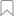Evaluate expressionTaraf Soulver | Güncelleyen 3 months ago | Other
Popülerlik

Gecikme

Hizmet Düzeyi

Evaluate expression Ürününe Genel Bakış

Takipçi Sayısı: 0
API Üreticisi:Soulver
soulver
API'yi Değerlendirin:
API'yi Değerlendirme İçin Giriş Yapın

Scans a natural language input and evaluates any valid mathematical expression found.

It can be used for many applications:

• Basic arithmatic:
eg: "5 + 450"
eg. “90k - 10%”

• Calendar calculations (working out dates & times)
Eg. "March 12 + 3 weeks"
Eg. "7:45am + 2 hours 25 minutes"
Eg. “July 30 to August 20 in days”

• Time zone conversions
Eg. "time in Tokyo"
Eg. "time difference between Chicago and Sydney"
Eg. “10am Paris to Auckland”

• Financial & percentage functions:
Eg: "25% of 900"
eg. "50 is 30% of what"
eg. “\$50k over 5 years at 7%” (compound interest)

• Unit conversions:
eg. "59 kg in pounds"
eg. “300 miles/hour in km/hour”

• Currency conversions (166 real-world currencies + 20 popular crypto-currencies supported):
eg. "20 USD in AUD"
eg. “50 Bitcoin in Euros”

eg: "random number between 10 and 25"
eg. “5 is to 50 as what is to 100” (answer = 10)
eg. “November 12 3:30pm as timestamp” (answer = 1605155400)

Here are some examples of what this API will return, given an expression.

Simple Arithmetic
1 + 1 (= 2)
25 − 5 (= 20)
9 × 9 (= 81)
20/5 (= 4)
56 ÷ 8 (= 7)
9^2 (= 81)

Mod / Remainder

Use the ‘mod’ operator to get the leftover number after a division

21 mod 5 (= 1)
remainder of 21 divided by 5 (= 1)

Percentage Operations

\$50 + 10% sales tax (= \$55.00)
\$120 − 30% discount (= \$84.00)

10% of 100 (= 10)
10% off 100 (= 90)
10% on 100 (= 110)

10 is what % of 200 (= 5%)
90 is what % off 100 (= 10%)
15 is what % on 10 (= 50%)

20 is 10% of what (= 200)
45 is 50% off what (= 90)
110 is 10% on what (= 100)

SoulverCloud supports many commonly used units of space & time, mass, temperature, volume and digital storage

Convert between units using ‘in’ or ‘to’:

12 hours in minutes (= 720 min)
100 km in miles (= 62.1371192237 mi)
32 C to F (= 89.6 °F)
170 lb in kg (= 77.11064 kg)
120 MB to GB (= 0.12 GB)
minutes in 4 days (= 5,760 min)

100 EUR in USD (= 109.05 USD)
¥30 in € (= €3.86)
10 BTC in Euros (= €84,362.53)

5 h 10 m in s (= 18,600 s)
1:4:30 + 0:25:50.5 (= 01:30:20.5)

When adding up units of different kinds, the answer will be shown in terms of the largest unit used
3 km + 10 m (= 3.01 km)

Or if the units do not share a common base unit, the last unit used
€30 + \$20 (= \$70.05)

Date & Time Calculations

10 June + 3 weeks 4 days (= 5 July)
April 1, 2019 − 3 months 5 days (= 27 December 2018)
9:35 am − 7 hours 15 minutes (= 2:20 am)
12/02/1988 + 32 years (= 12 February)
01.05.2005 + 3 years 2 months 3 weeks (= 22 July 2008)

March 20 to June 5 (= 2 months 2 weeks 2 days)
17:30 to 20:45 (= 3 hours 15 minutes)
June 12 to July 15 in days (= 33 days)
weeks between October 21 and December 2 (= 6 weeks)
months from Feb 12 to Sep 9 (= 6 months)

days since December 1 (= 175 days)
weeks before March 8 (= 41 weeks)

workdays between January 4 and January 8 (= 4 workdays)
June 12 to June 19 in workdays (= 5 workdays)

midpoint between 30 and 90 (= 60)
half of 500 (= 250)

lesser of 5 and 10 (= 5)
greater of 50 and 40 (= 50)

6 is to 60 as 8 is to what (= 80)
5 is to 10 as what is to 80 (= 40)

random number between 1 and 10 (= 2)
random number between 0 and 1 (= 0.005212715)

Değerlendirme: 5 - Oy Sayısı: 1